# Logarithm - math word problems

#### Number of problems found: 49

• LogarithmDetermine the number whose decimal logarithm is -3.8.
• Seating rules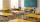In a class are 24 seats but in 7.B class are only 18 students. How many ways can student seat? (The class has 12 benches. A bench is for a pair of students.) Result (large number) logarithm and thus write down as powers of 10.
• Logif ?, what is b?
• Exp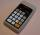If ?, then n is:
• Powers 3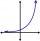2 to the power of n divided by 4 to the power of -3 equal 4. What is the vaule of n?
• SequenceCalculate what member of the sequence specified by ? has value 86.
• Log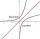Calculate value of expression log |3 +7i +5i2| .
• Exponential equationSolve for x: (4^x):0,5=2/64.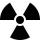After 548 hours decreases the activity of a radioactive substance to 1/9 of the initial value. What is the half-life of the substance?
• Exponential equationIn the set R solve the equation: ?
• Logarithmic equation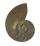Solve equation: log33(3x + 21) = 0
• What isWhat is the annual percentage increase in the city when the population has tripled in 20 years?
• Intercept with axisF(x)=log(x+4)-2, what is the x intercept
• Gain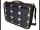Find the gain whenever the output power is 21x the input power.
• Dice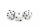How many times must throw the dice, the probability of throwing at least one six was greater than 90%?
• Car valueThe car loses value 15% every year. Determine a time (in years) when the price will be halved.
• Compound interestCalculate time when deposit in the bank with interest 2.5% p.a. doubles.
• Coordinate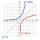Determine missing coordinate of the point M [x, 120] of the graph of the function f bv rule: y = 5x
• An investmentHow long will it take for an investment to double at a simple interest rate of 5.10% p. A. ? Express the answer in years and months, rounded up to the next month.
• Sum-log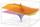The sum of two numbers is 32, the sum of their logarithms (base 10) is 2.2. Determine these numbers.

Do you have an interesting mathematical word problem that you can't solve it? Submit a math problem, and we can try to solve it.

We will send a solution to your e-mail address. Solved examples are also published here. Please enter the e-mail correctly and check whether you don't have a full mailbox.

Please do not submit problems from current active competitions such as Mathematical Olympiad, correspondence seminars etc...